# Easiest colleges to get into in Maryland

Top 10 colleges in Maryland with the highest acceptance rates
What are the easiest colleges to get into in Maryland? We've got you covered. We've compiled a national college database and have created a list of the easiest colleges to get into in Maryland below. These are the easiest 4 year colleges to get into in Maryland that have the highest acceptance rates, and are more likely to accept low GPAs, GEDs, and everything in between. There are tons of good colleges below that accept more applicants, and we also share their average SAT and ACT scores so that you can see where you're most competitive. Read on to find out more.

## McDaniel College acceptance rate

McDaniel College acceptance rate is 85%.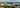The average SAT score for McDaniel College is 1154.

• SAT composite: 1154
• SAT math: 570

The average ACT score for McDaniel College is 24.

## Saint Mary's College of Maryland acceptance rate

Saint Mary's College of Maryland acceptance rate is 80.1%.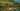The average SAT score for Saint Mary's College of Maryland is 1180.

• SAT composite: 1180
• SAT math: 580

The average ACT score for Saint Mary's College of Maryland is 25.

## Goucher College acceptance rate

Goucher College acceptance rate is 79.2%.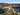The average SAT score for Goucher College is 1200.

• SAT composite: 1200
• SAT math: 570

The average ACT score for Goucher College is 25.

## Towson University acceptance rate

Towson University acceptance rate is 76.3%.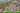The average SAT score for Towson University is 1140.

• SAT composite: 1140
• SAT math: 560

The average ACT score for Towson University is 23.

## Washington College acceptance rate

Washington College acceptance rate is 74.4%.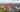The average SAT score for Washington College is 1180.

• SAT composite: 1180
• SAT math: 580

The average ACT score for Washington College is 26.

## Frostburg State University acceptance rate

Frostburg State University acceptance rate is 72.3%.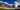The average SAT score for Frostburg State University is 1060.

• SAT composite: 1060
• SAT math: 520

The average ACT score for Frostburg State University is 20.

## Hood College acceptance rate

Hood College acceptance rate is 71.4%.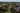The average SAT score for Hood College is 1160.

• SAT composite: 1160
• SAT math: 570

The average ACT score for Hood College is 24.

## Loyola University Maryland acceptance rate

Loyola University Maryland acceptance rate is 65.5%.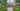The average SAT score for Loyola University Maryland is 1240.

• SAT composite: 1240
• SAT math: 620

The average ACT score for Loyola University Maryland is 27.

## Maryland Institute College of Art acceptance rate

Maryland Institute College of Art acceptance rate is 64.3%.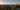The average SAT score for Maryland Institute College of Art is 1208.

• SAT composite: 1208
• SAT math: 600

The average ACT score for Maryland Institute College of Art is 25.

## Mount Saint Mary's University acceptance rate

Mount Saint Mary's University acceptance rate is 64.2%.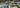The average SAT score for Mount Saint Mary's University is 1080.

• SAT composite: 1080
• SAT math: 530